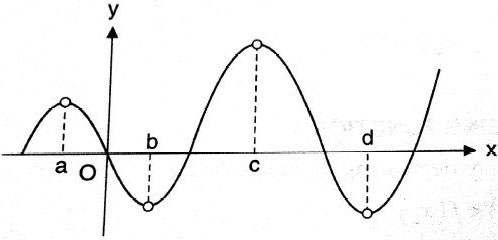Q

# Help me understand! Which of the following graphs represents local minima at x = a ?

Which of the following graphs represents local minima at x = a ?

• Option 1)• Option 2)• Option 3)• Option 4)145 Views

As we have learned

Local maximum and Local minimum -

Let  y = f(x)  be the given function then     is a point of local maximum if there exists an open interval containing    such that

for all values of  x  lying in that interval and for   then it is local minimum in fig and at

- whereinHere (D) is the only case where we will have an interval in the neighbourhood of x= a which contains x=a and

Option 1)Option 2)Option 3)Option 4)Exams
Articles
Questions